# AP Physics 1 Multiple-Choice Practice Test 24

### Test Information10 questions18 minutes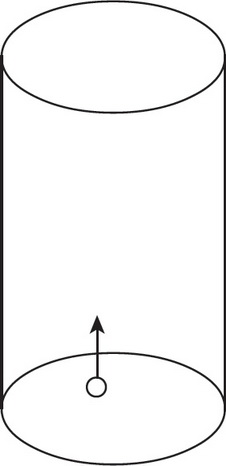1. A bubble in a glass of water releases from rest at the bottom of the glass and rises at acceleration a to the surface in t seconds. How much farther does the bubble travel in its last second than in its first second? Assume that the journey takes longer than 2 seconds.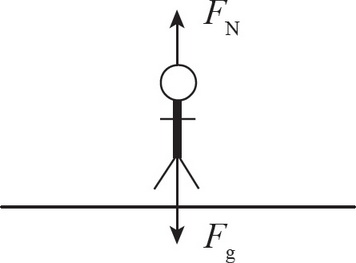2. A person standing on a horizontal floor is acted upon by two forces: the downward pull of gravity and the upward normal force of the floor. These two forces

3. Which of the following graphs best represents the force of friction on an object starting at rest that eventually starts sliding across a level surface due to a gradually increasing horizontal force?

4. Two objects have a mass 1 kg and carry a charge of magnitude 1 C each. Which statement correctly identifies the relationship between the magnitude of the force of gravity, Fg, and the magnitude of the electric force, FE, between the objects?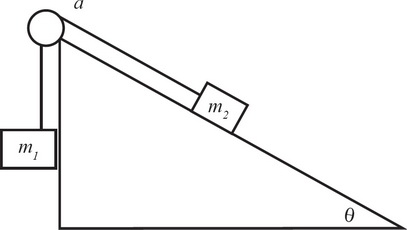5. Consider the above configuration of masses attached via a massless rope and pulley over a frictionless inclined plane. What is the acceleration of the masses?

6. A person is pulling a block of mass m with a force equal to its weight directed 30° above the horizontal plane across a rough surface, generating a friction f1 on the block. If the person is now pushing downward on the block with the same force 30° below the horizontal plane across the same rough surface, what is the friction on the block? (μk is the coefficient of kinetic friction across the surface.)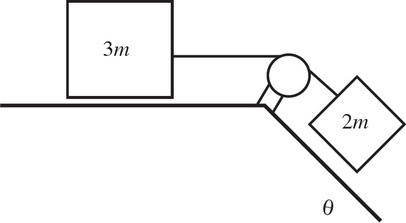7. In the figure above, two blocks of mass 3m and 2m are attached together. The plane is frictionless and the pulley is frictionless and massless. The inclined portion of the plane creates an angle θ with the horizontal floor. What is the acceleration of the block 2m if both blocks are released from rest (gravity = g)?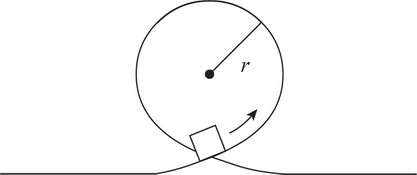8. If a roller coaster cart of mass m was not attached to the track, it would still remain in contact with a track throughout a loop of radius r as long as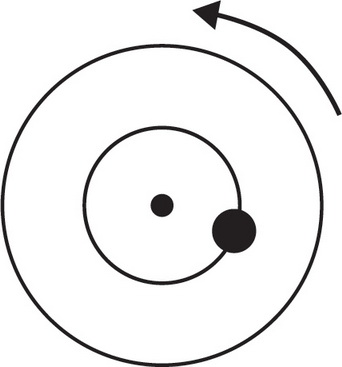9. The diagram above shows a top view of an object of mass M on a circular platform of mass 2M that is rotating counterclockwise. Assume the platform rotates without friction. Which of the following best describes an action by the object that will increase the angular speed of the entire system?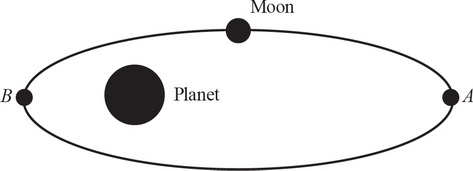10. A moon has an elliptical orbit about the planet as shown above. The moon's mass is much smaller than the planet's. Which of the following quantities is/are (approximately) conserved as the moon moves from point A to point B ?

I.The moon's mechanical energy

II.The moon's angular momentum

III.The moon's (linear) momentum